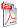Issue archive

https://doi.org/10.15255/KUI.2019.024
Published: Kem. Ind. 69 (3-4) (2020) 111–127
Paper reference number: KUI-24/2019
Paper type: Original scientific paper
Download paper:PDFA Comparison of “Neural Networks and Multiple Linear Regressions” Models to Describe the Rejection of Micropollutants by Membranes

Y. Ammi, L. Khaouane and S. Hanini

##### Abstract

A rejection process of organic compounds by nanofiltration and reverse osmosis membranes was modelled using the artificial neural networks. Three feed-forward neural networks based on quantitative structure-activity relationship (QSAR-NN models) characterised by a similar structure (twelve neurons for QSAR-NN1, QSAR-NN2, and QSAR-NN3 in the input layer, one hidden layer and one neuron in the output layer), were constructed with the aim of predicting the rejection of organic compounds. A set of 1394 data points for QSAR-NN1, 980 data points for QSAR-NN2, and 436 data points for QSAR-NN3 were used to construct the neural networks. Good agreements between the predicted and experimental rejections were obtained by QSAR-NN models (the correlation coefficient for the total dataset were 0.9191 for QSAR-NN1, 0.9338 for QSAR-NN2, and 0.9709 for QSAR-NN3). Comparison between the feed-forward neural networks and multiple linear regressions based on quantitative structure-activity relationship “QSAR-MLR” revealed the superiority of the QSAR-NN models (the root mean squared errors for the total dataset for the QSAR-NN models were 10.6517 % for QSAR-NN1, 9.1991 % for QSAR-NN2, and 5.8869 % for QSAR-NN3, and for QSAR-MLR models they were 20.1865 % for QSAR-MLR1, 19.3815 % for QSAR-MLR2, and 16.2062 % for QSAR-MLR3).

##### Keywords

neural networks, multiple linear regressions, models, rejection, micropollutants, membranes